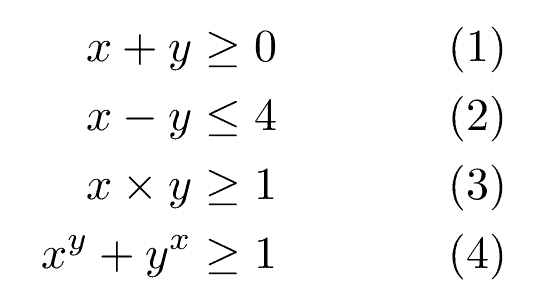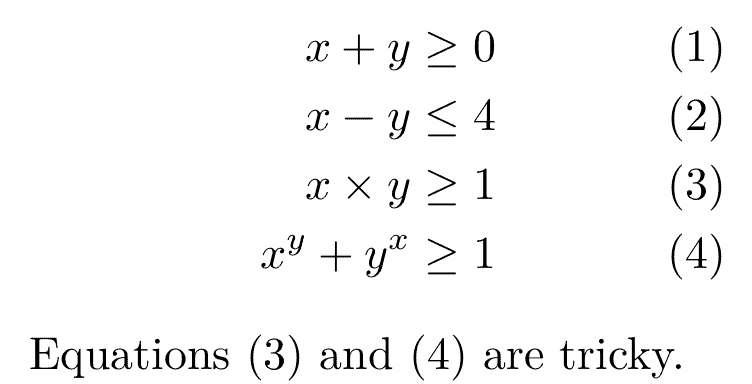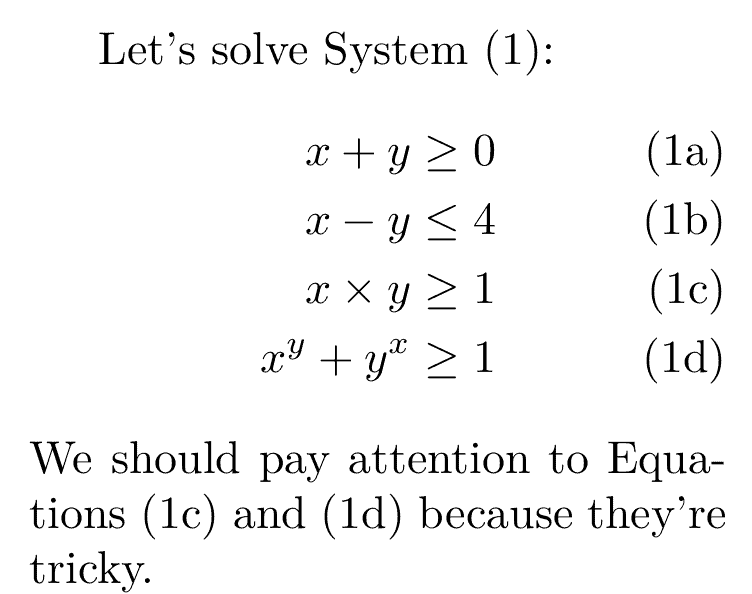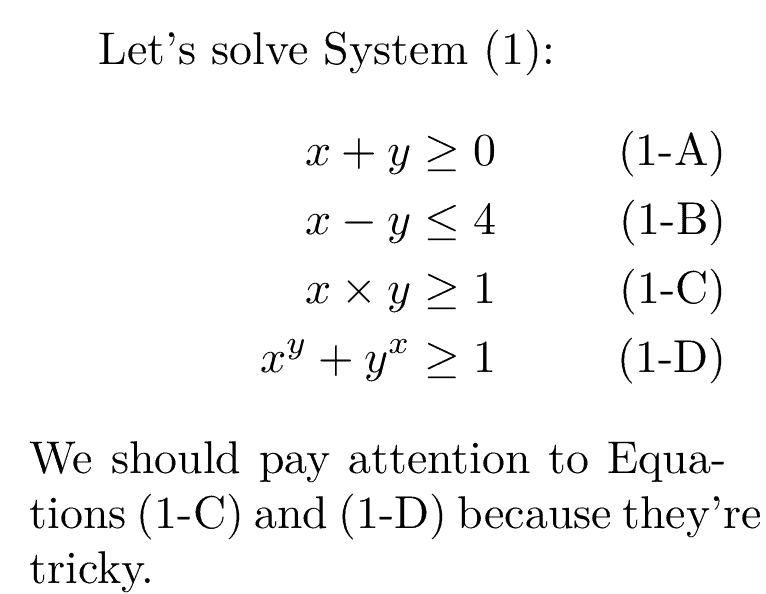## 1. Introduction

In this tutorial, we’ll talk about referencing subordinate equations in LaTeX.

## 2. The Problem Statement

Sometimes, we need to show a system of equations. There are plenty of ways to do that. For instance, we can use the align environment from the amsmath package:

\begin{align}
x + y & \geq 0 \\
x - y & \leq 4 \\
x \times y & \geq 1 \\
x^y + y^x & \geq 1
\end{align}

We get:We can label each equation and reference it later in the text:

\begin{align}
x + y & \geq 0 \label{eq:sum} \\
x - y & \leq 4 \label{eq:difference}\\
x \times y & \geq 1 \label{eq:product}\\
x^y + y^x & \geq 1 \label{eq:exp_sum}
\end{align}
Equations \eqref{eq:product} and \eqref{eq:exp_sum} are tricky.

The result is:However, the equation numbers don’t show that the corresponding equations are parts of the same system. It would be better if the numbers were (1a), (1b), (1c), and (1d).

Additionally, we can’t reference the system as a whole with a single reference number. Following this approach, we always have to reference all the equations or at least the start and end ones, which is cumbersome.

## 3. The subequations Environment

This subequations environment solves both problems. It allows us to reference the entire system and individual equations:

Let's solve System \eqref{eq:system}:
\begin{subequations} \label{eq:system}
\begin{align}
x + y & \geq 0  \label{eq:sum} \\
x - y & \leq 4 \label{eq:diff}\\
x \times y & \geq 1 \label{eq:prod} \\
x^y + y^x &\geq 1 \label{eq:exp_sum}
\end{align}
\end{subequations}
We should pay special attention to Equations \eqref{eq:prod} and \eqref{eq:exp_sum} because they're tricky.

The result is:The \label right after \begin{subequations} is for the entire system. It’s one plus the number of the previous numbered equation. For example, if the previous equation’s label was (2), the one for the system would be (3).

The subordinate labels are formed by enumerating the equations within the system. By default, the environment uses lowercase Latin letters, but we can change that.

### 3.1. Customizing Subordinate Labels

The counter used for subordinate equations is equation. The command that prints the counter is \theequation. Knowing that the entire system’s label is produced by \theparentequation, we can format the display of equation by renewing the \theequation command.

For example, we may want to show the subordinate labels as (1-A), (1-B), and so on. To do so, we format their display using the \Alph command inside the subequations environment:

\renewcommand{\theequation}{\theparentequation-\Alph{equation}}

and get this:We can use other numbering systems instead of \Alph. Here’s what LaTeX natively supports:

• \roman – lowercase Roman numerals
• \Roman – uppercase Roman numerals
• \arabic – Arabic numerals
• \alph – lowercase Latin letters
• \Alph – uppercase Latin letters

Further, if we want the labels to be clickable hyperlinks, we can use the hyperref package.

## 4. Conclusion

In this article, we showed how to reference subordinate equations in LaTeX and customize their labels.